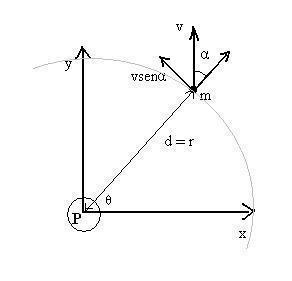# Angular Diameter

The apparent size or angular diameter refers to an object’s size as viewed from a specified place. It is the object’s visual diameter as determined from an angle. This is known as a visual angle. It is the visual diameter perspective projection diameter of an object. In this case the object is set on a plane through its middle that is perpendicular to the view direction.

### Apparent Sizes of the Earth and SunThe apparent size of the orbit of the Earth round the Sun is 2” (two arcseconds). This is from a one parsec distance. The apparent size of the Sun is 0.03”, and the Earth 0.0003”. This is at a light year distance. The Sun 0.03” apparent size is similar to that of an individual at a distance of the Earth’s diameter.

### Apparent Sizes of Celestial Bodies

These angular sizes are based on views on Earth. For the Sun it is 31.6’ – 32.7’; for the Moon it is 29.3’ – 34.1’ and for Venus it is 9.565” – 66.012”. The angular diameter of Jupiter is 29.800” – 50.115” and for Saturn it is 14.991” – 20.790”. For Mars the apparent size is 3.492” – 25.113”. The apparent size of Mercury is 4.535” – 13.019”.

The apparent size of Uranus is 3.340” – 4.084”. Neptune’s apparent size is 2.179” – 2.373”. Ceres is 0.330” – 0.840”. The apparent size of Vesta is 0.20” – 0.64”. For Proxima Centauri it is 0.001”. For Deneb it is 0.002”. The apparent size of Altair is 0.003”.

The apparent size of Sirius is 0.005936” and for Canopus it is 0.006”. For Alpha Centauri A, it is 0.007” and for Alphard it is 0.00909”. The apparent size of Eris is 0.00909”. Betelgeuse is 0.049” – 0.060”; R Doradus has an apparent size of 0.052” – 0.062” and for Pluto it is 0.063” – 0.115”.

### More about the Sun Apparent Size

The figures given indicate the Sun apparent size is 250,000 times compared to Sirius. The Sun apparent size is 250,000 compared to Alpha Centauri A. The Sun is 40 million times as bright. The Sun’s apparent size is almost equal to the Moon in this respect. Objects that are not circular are given two diameter measurements: Minor and Major Diameters. For instance, the Small Magellanic Cloud has a 5 degree 20’ x 3 degree 5’ visual diameter.

Angular diameter measurements may not be the same as the object’s physical diameter because of foreshortening. The figures are the same for a disc shaped object however.